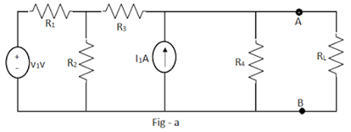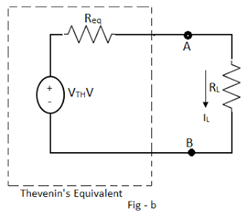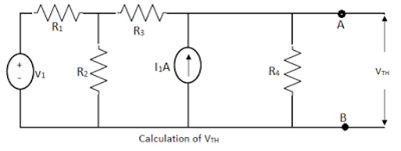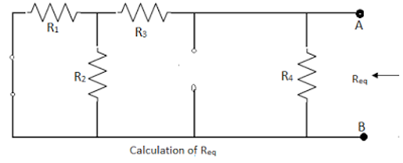# Explanation of Thevenin's Theorem

### Statement of Thevenin’s Theorem

Any permutation of linear bilateral circuit elements and active sources, apart from the connections or complication, connected to a certain load RL possibly will replace by a simple two terminal network consisting of a single voltage source of Vm volts and a single resistance Req in series with the voltage source, across the two terminals of the resistance load RL. The voltage VTH is the open circuit voltage measured at the two terminals of interest, with load resistance RL removed. This voltage is known as Thevenin's equivalent voltage. The Req is the equivalent resistance of the given network as viewed through the terminals where RL is connected, but with RL removed and all the active sources are replaced by their internal resistances.

### Explanation of Thevenin's Theorem

The theory of Thevenin's equivalent across the terminals of interest can be explained by considering the circuit shown in the Fig-a. The terminals A-B are the terminals of interest across which RL is connected. Then Thevenin's equivalent across the load terminals A-B can be obtained as shown in the Fig-b.The voltage VTH is obtained across the terminals A-B with RL removed. Hence VTH is also called open circuit Thevenin's voltage. The circuit to be used to calculate VTH is shown in the Fig-a, for the network considered above. While Req is the equivalent resistance obtained as viewed through the terminals A-B with RL removed, voltage sources replaced by short circuit and current sources by open circuit. This is shown on the fig - b.While obtaining VTH, any of the network simplification techniques can be used. When the circuit is replaced by Thevenin's equivalent across the load resistance, then the load current can be obtained as

IL = VTH/(RL + Req).

By using this theorem, current through any branch of the circuit can be obtained, treating that branch resistance as the load resistance and obtaining Thevenin's equivalent across the two terminals of that branch resistance as the load resistance and obtaining Thevenins equivalent across the two terminals of the branch.

#### Steps to Apply Thevenin's Theorem

1. Remove the branch resistance through which current is to be calculated.
2. Calculate the voltage across these open circuited terminals, by using any of the network simplification techniques.  This is VTH.
3. Calculate Req as viewed through the two terminals of the branch from which current  is to be calculated by removing that branch resistance and replacing all independent sources by their internal resistances. If the internal resistances are not known then replace Independent   voltage sources by short circuits and independent current sources by open circuits.
4. Draw the Thevenin's equivalent showing source VTH, with the resistance Req in series with it, across the terminals of branch of interest.
5. Reconnect the branch resistance. Let it be RL. The required current through the branch is given by,
I = VTH / (Req + RL)

#### Limitations of Thevenin's Theorem:

The limitations of Thevenin's theorem are;
1. Not applicable to the circuits consisting of nonlinear elements.
2. Not applicable to unilateral networks.
3. There should not be magnetic coupling between the load and circuit to be replaced by Thevenin's theorem.
4. In the load side, there should not be controlled sources, controlled from some other part of the circuit.0 CommentsComments